# Simple equation

Solve for x:

3(x + 2) = x - 18

Correct result:

x =  -12

#### Solution:

3(x + 2) = x - 18

3•(x + 2) = x - 18

2x = -24

x = -12

Our simple equation calculator calculates it.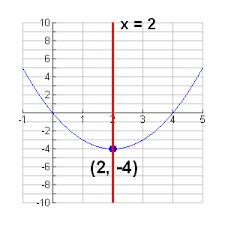We would be pleased if you find an error in the word problem, spelling mistakes, or inaccuracies and send it to us. Thank you!Tips to related online calculators
Do you have a linear equation or system of equations and looking for its solution? Or do you have quadratic equation?

## Next similar math problems:

• Soccer balls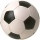Pupils in one class want to buy two soccer balls together. If each of them brings 12.50 euros, they will miss 100 euros, if each brings 16 euros, they will remain 12 euros. How many students are in the class?
• The string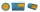They cut 113 cm from the string and divided the rest in a ratio of 5: 6.5: 8: 9.5. The longest part measured 38 cm. Find the original length of the string.
• Trip cost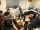In September, the trip cost CZK 12,000. How many crowns did the trip cost in June of the same year, when they have since reduced the price by a quarter and by another CZK 1,200?Susan thought, "If I read 15 pages a day, I will read the whole book in 8 days. “How many pages would she have to read a day if she wanted to finish the book on the 6th day from the start of reading? And how many pages does the book have?
• Worker salaryThe worker had a salary of CZK 18,000. During the year, his salary was increased by a quarter. He earned a total of CZK 247,500 for the whole year. From which month was his salary increased?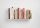If I read 15 pages a day, I will read the whole book in 18 days. How long will it take to read a book if I only read 9 pages every day?
• Sum of sevenThe sum of seven consecutive odd natural numbers is 119. Determine the smallest of them.
• The sumThe sum of five consecutive odd numbers is 75. Find out the sum of the second and fourth of them.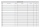Suzan reads a book. If she read half an hour a day, she would read it in nine days. How many minutes does he have to read a day if he wants to read it three days earlier?
• The tourist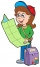The tourist walked a quarter of the way on the first day, a third of the rest on the second day, and 20 km on the last day. How many km did he walk in three days?
• What time is it?What time is it, when there is 4 times less time left until midnight than the time that has elapsed since noon? What time is it, when one-fifth of the hours that have passed since midnight is equal to one-third of the hours that are missing by 12 o'clock?
• Second sideCalculate the length of the other side of the rectangle if its circumference is 60 cm and one side is 10 cm long.
• Work togetherTwo bricklayers plastering a wall. The first would plaster it in 8 hours, the second in 12 hours. How many hours will they be done with the work if they work together?
• DiggersThe excavation was carried out in 4 days and 5 workers worked on it for 7 hours a day. Determine how long it would take to excavate if 7 workers worked on it 8 hours a day.
• Tourist routeHow long is the tourist route when tourists crossed four-sevenths of the way on foot, crossed the bus twice less than on foot and passed the last 14 kilometers by boat.
• The farmerThe farmer calculated that the supply of fodder for his 20 cows was enough for 60 days. He decided to sell 2 cows and a third of the feed. How long will the feed for the rest of the peasant's herd last?
• Job applicantsJob applicants: three-fourths of applicants had experience for a position. The number that did not have prior experience was 36. How many people applied for the job?